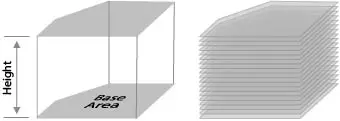# Irregular Prism Volume Calculator

This Irregular Prism Volume Calculator is designed to help you determine the volume of an irregular prism.

To use the calculator, simply enter the base area and height of the prism and click "Calculate". As you enter new dimensions into the volume calculator, a running total will also be displayed.

## Basic definition and general information about an Irregular Prism

A prism is said to have the prism's cross sections running all along its length. The diagram below is used to illustrate an example of an irregular prism that has been divided into sections. It is important to note that each cross section of an irregular prism disposes of the same dimensions and area.The basic definition of an irregular prism would be a figure that does not comply with the general mathematical rules.

The following formula is used to calculate the volume of an irregular prism:

• Volume = Base Area × Height

Note: Units are provided for convenience purposes only and do not have any impact on the calculations.

Units used for measuring the volumes of solid objects are as follows:

• cubic feet
• cubic meters
• cubic yards

Units used to measure the volumes of liquids are:

• liters
• quarts
• pints
• gallons